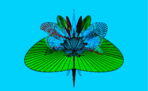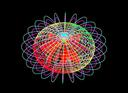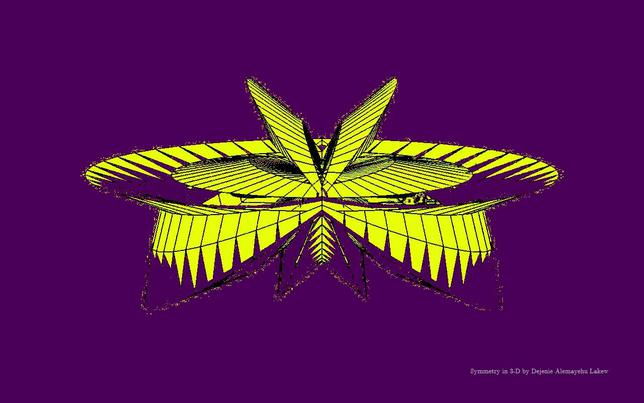This  is a mathematical website
It presents mathematics, mathematical ideas
and reflections on mathematics.

 HOMEAbout meCoursesLinksColumnGalleryPublicationsAMS JournalsMAA JournalsDe Gruyter JournalsarXiv.orgEJCSM: E-Journal(∀∈>0)(∃δ>0):∣x-x₀∣ < δ ⇒ ∣ψ(x)-ψ(x₀)∣ < ∈All images on this page are compositions of 2-D surfaces in 3-D space with several tilts and turns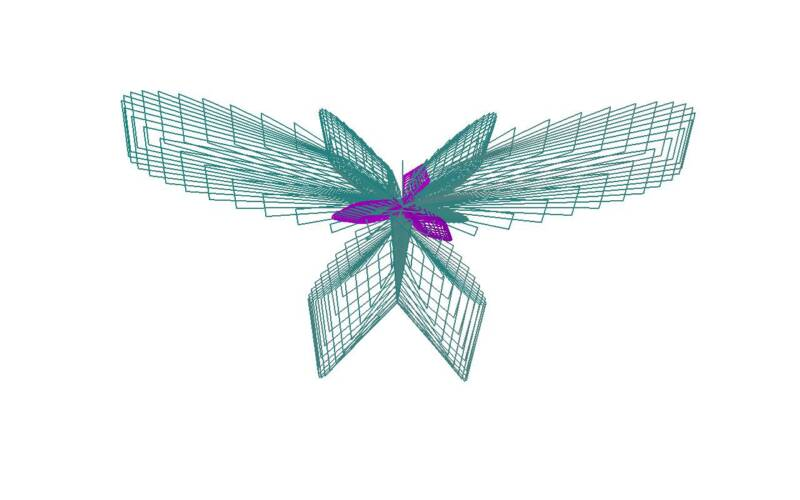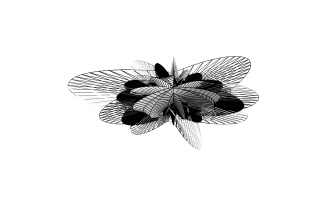You are the                Visitor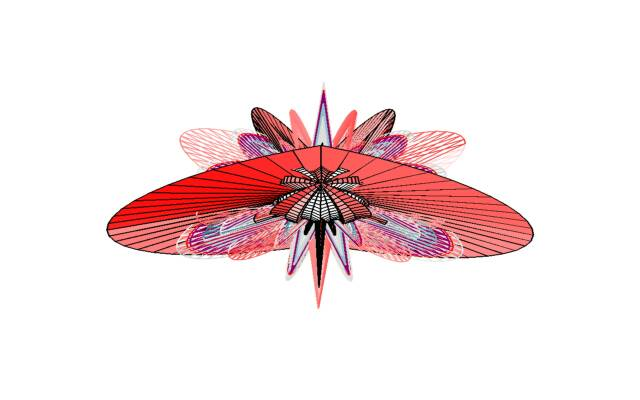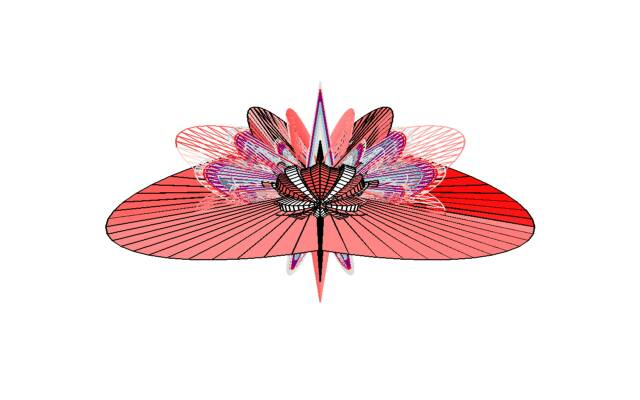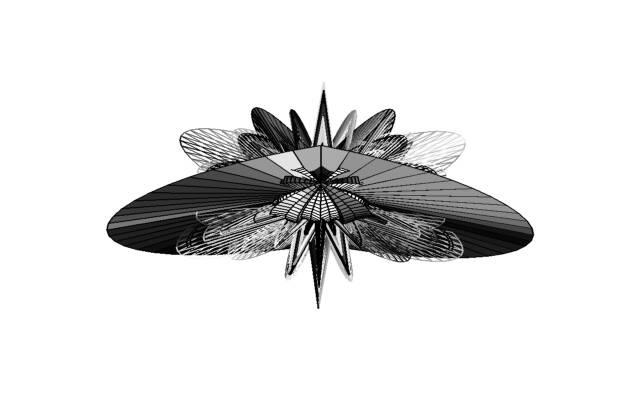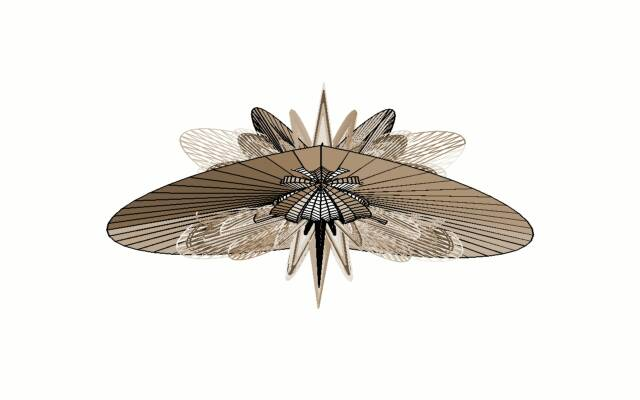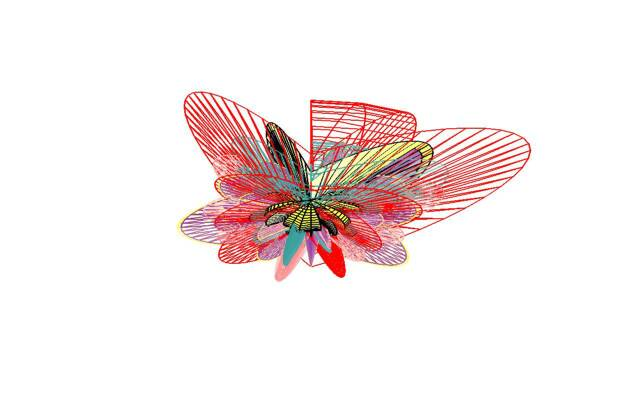" MORE THAN 90% OF TOOLS IN GOD'S WORKSHOP ARE MATHEMATHICAL ".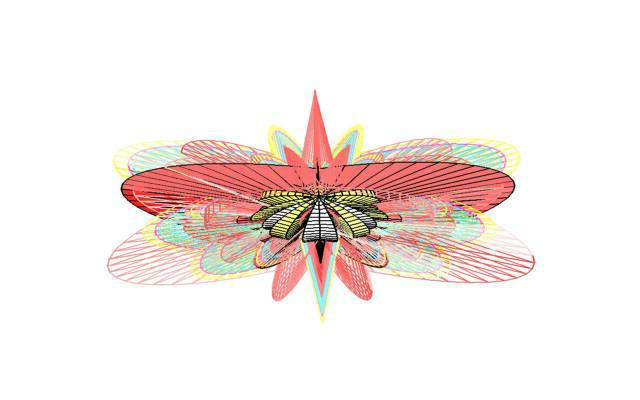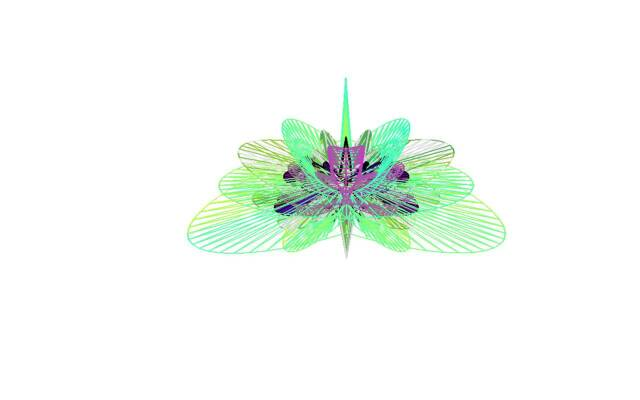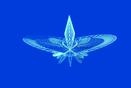M
A
T
H
E
M
A
T
I
C
A
L

I
M
A
G
E
S
M
A
T
H
E
M
A
T
I
C
A
L

I
M
A
G
E
S
HYPER SYMMETRIES-MATHEMATICAL IMAGERY
BY DEJENIE A. LAKEW,PH.D.
AUTHORHOUSE, 2009
Available also at Major Bookstores :
Amazon, Barnes & Nobles, Books-A-Million

It is a book of mathematical images. It can be a gift to your kids, friends or to your self. You will enjoy the images.
M
A
T
H
E
M
A
T
I
C
A
L

I
M
A
G
E
S
M
A
T
H
E
M
A
T
I
C
A
L

I
M
A
G
E
S

My New Mathematical Identities (Verify them) on a new kind of
differential operator I defined by:  ∑_{k=0}^{∞}((D^{(k)}/(k!)

1.  ∑_{k=0}^{∞}((D^{(k)}(cos x))/(k!)) = cos(x+1)
2.  sin x+∑_{k=1}^{∞}((D^{(k)}(sin x))/(k!)) = sin(x+1)
3.  ∑_{k=0}^{∞}((D^{(k)}(e^ (x))/(k!)) = e^(x+1)

- Dejenie Alemayehu Lakew

More new results and conjectures on the new operator
that I defined on the left are posted on my
ResearchGate site in Math group under the category:

Communications in Mathematics Teaching:CMT

Check it out ! I welcome comments or counter
examples on the conjectures.Definite Integral :

lim ∑{k=0}ⁿ =∫_{a}^{b}
n→∞
M
A
T
H
E
M
A
T
I
C
A
L

I
M
A
G
E
S
M
A
T
H
E
M
A
T
I
C
A
L

I
M
A
G
E
S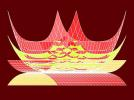e^{ikπ}±1=0
ℝ   ℂ   Cl_{n}
ℕ   W   ℤ   ℚ

Sett of Numbers: Natural to rationals
Set of Numbers: Reals to CliffordMath'l Symbols

∈,⊆,⊇
∪,∩,∋
+, -,×,÷,±
=,≡, ≤, ≥
⇒,⇐,⇔
∨,∧,∼
⇉,⊥,∥
∑, ∏ , ∫

∴, ∵, ≅
MATHEMATICAL IMAGES OF SYMMETRY AND HYPER-SYMMETRYMathematics is both useful and  beautiful
Les Mathematiques sont dueux utiles et beauMathematica est utilis et pulchra
МАТЕМАТИКА одновременно полезные и красивые
מתמטיקה הוא גם שימושי ויפה
الرياضيات هي مفيدة وجميلة
Μαθηματικά είναι τόσο χρήσιμο και όμορφοMathematik ist sowohl nützlich als auch beautiful
ሂሳብ በጣም ጠቃሚና ውበት ያለው የጥናትና የምርምር መስክ ነው∫_{Ω}φ(((∣u∣)/λ))dx < ∞MATHEMATICS CONDITIONS US HOW WE THINK AS TECHNOLOGY DOES HOW WE LIVE - DEJENIE ALEMAYEHU LAKEW
∄A:ℵ₀<∣A∣<2^{ℵ₀}
∑ → ∫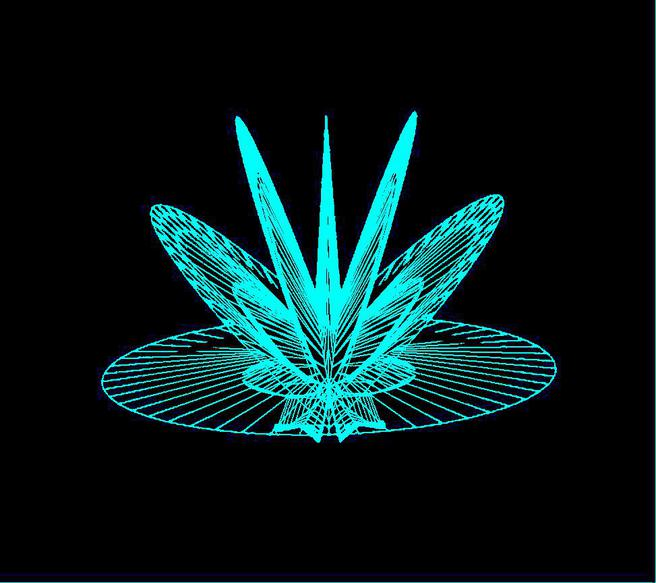MATHEMATICS
π{ψ_{k}:k∈ℤ⁺}
数学是既有用又美观
수학은 유용하고 아름다운 둘 다
数学は便利で美しい両方です
गणित उपयोगी और सुंदर दोनों है
Wiskunde is beide nuttig en mooi
ریاضیات هر دو مفید و زیبا است

"A mathematician, like a painter or poet, is a maker of patterns. If his patterns are  more permanent than theirs, it is because they are made with ideas"
~   G. H. Hardy

​Constellation of Analysis

M
A
T
H
E
M
A
T
I
C
A
L

A
R
T
Art and Mathematics
"Mathematics has to be studied not only because it is useful, but because
it is also beautiful, as it is a study of structures of logic and reason that   transcends the expanse of imagination"
~  Dr. Dejenie Alemayehu Lakew
The Galaxy of Mathematics
Constellation of Algebra
Constellation of Geometry
Gravity of Logic
Dark Matter  of Imagination
Stars of Arithmetic and Number Theory
Stars of Algebraic and Differential Geometry
Stars of Topology and Algebraic Topology